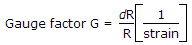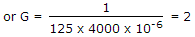# Electronics and Communication Engineering - Measurements and Instrumentation

### Exercise :: Measurements and Instrumentation - Section 2

1.

Kelvin's double bridge is used to measure low resistances because

 A. it has high sensitivity B. there is no thermoelectric emf C. resistance variation due to temperature D. effect of contact and lead resistances is eliminated

Explanation:

Effect of lead and contact resistances is very important in measurement of low resistances.

2.

Assertion (A): Shunt of an ammeter has a low resistance.

Reason (R): Shunt may be connected in series or in parallel with ammeter.

 A. Both A and R are true and R is correct explanation of A B. Both A and R are true but R is not correct explanation of A C. A is true R is false D. A is false R is true

Explanation:

Shunt is always connected in parallel with ammeter.

3.

The scale of hot wire instrument is linear.

 A. True B. False

Explanation:

Since reading is proportional to I2, the scale is non-linear.

4.

The D'Arsonval- movement can be converted into audio frequency ac ammeter by adding a

 A. thermocouple B. transducer C. chopper D. rectifier

Explanation:

Rectifier converts ac to dc which is measured by D'Arsonval meter.

5.

The resistance of a 125 Ω strain gauge changes by 1 ohm for 4000 micro strain. The gauge factor is

 A. 1.5 B. 2 C. 2.5 D. 3

Explanation:.